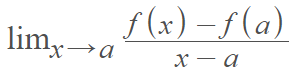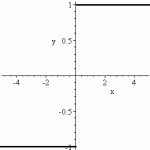# Derivative Does Not Exist at a Point: 7 Examples

## Derivative Does Not Exist at a Point

The derivative at a point exists when the limit at the point exists:Therefore, if the limit doesn’t exist, then the derivative doesn’t exist either. There are two general situations where this might happen:

1. When the curve doesn’t have a tangent line because of a discontinuity or sharp corner.
2. When the tangent line has an infinite slope (i.e. there is a vertical inflection point).

## 7 Examples of When the Derivative Does Not Exist at a Point

There are a few specific situations that causes a curve not to have a tangent line or an infinite slope:

1. Jump discontinuity: a gap in a graph means that the function is not continuous and therefore not differentiable.This graph jumps at the origin. The derivative does not exist at this point (x = 0).
2. Hole in the graph: Holes (more formally called removable discontinuities) are tiny gaps in a graph. One example of when a hole happens is when a rational function has an x-value, that when plugged into the function causes both the numerator and the denominator to equal 0.
3. Unbounded behavior or an infinite discontinuity. The x-values get larger and larger as you try and move towards the point in question.As you move towards the point x = 0 from the right, the y-values go towards infinity.
4. Sharp points or cusps make a function not differentiable at that point.A sharp corner, in this case at x = 0, means the derivative doesn’t exist at that point.
5. The function can’t be defined. For example, the square root function is not defined for values less than zero, so the derivative does not exist at any point less than zero.
6. The function can be defined but the derivative is infinity at the point in question (or it doesn’t exist at all). Derivatives equal to infinity happens quite often with rational functions and it means that there is a vertical tangent at that point
7. Oscillating behavior. Some functions behave badly and have oscillating discontinuities near certain points.The function sin(1/x) has an oscillating discontinuity at x = 0.

## References

Hole image created with Desmos.com.

CITE THIS AS:
Stephanie Glen. "Derivative Does Not Exist at a Point: 7 Examples" From StatisticsHowTo.com: Elementary Statistics for the rest of us! https://www.statisticshowto.com/derivative-does-not-exist-at-a-point-7-examples/